Latest Banking jobs   »   Quantitative Aptitude Quiz For ESIC- UDC,...

# Quantitative Aptitude Quiz For ESIC- UDC, Steno, MTS Prelims 2022-10th January

Q1. The ratio of numerical value of rate of interest and time period at a sum invested is 5 : 2 and men get 22.5% of invested sum as interest. Find at the same rate how much interest man will get on the sum of Rs. 1600 for two year ?
(a) 240 Rs.
(b) 180 Rs.
(c) 160 Rs.
(d) 120 Rs.
(e) 224 Rs.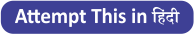Q2. Deepak and Ayush invested Rs. 12600 together in a business. Deepak invested for 8 months and Ayush invested for 15 months, if Deepak and Ayush get profit in the ratio of 75 : 128, then find the amount invested by Ayush ?
(a) 2400 Rs.
(b) 3000 Rs.
(c) 3600 Rs.
(d) 4800 Rs.
(e) 6400 Rs.

Q3. Mohit takes half the time to do a work as Ayush takes to complete the same work, while Veer takes same time as Ayush & Mohit takes to complete the work together. Find in how many days Veer will complete work together, if all three complete the work in 6 days ?
(a) 8 days
(b) 12 days
(c) 6 days
(d) 9 days
(e) 18 days

Q4. A vessel contains 96ℓ of mixture of milk and water in the ratio of 5 : 3 respectively. If 24ℓ of mixture taken out and some quantity of milk and water added in the ratio of 3 : 5 so the new ratio of milk and water becomes 15 : 13. Find quantity of water added ?
(a) 12.5 ℓ
(b) 15 ℓ
(c) 25 ℓ
(d) 7.5 ℓ
(e) 30 ℓ

Q5. The ratio between length of two trains is 2 : 3 and speed of these two trains is 108 km/hr and 96 km/hr respectively. if smaller train cross a 360 m long platform in 16 sec, then find the time taken by both trains to cross each other running in same direction ?
(a) 90 sec
(b) 84 sec
(c) 96 sec
(d) 108 sec
(e) 112 sec

Q6. The ratio between length of a rectangle and side of a square is 8 : 9. If breadth of rectangle is 10 cm and perimeter of square is 20 cm more than that of perimeter of rectangle, then find difference between area of square & that of rectangle?
(a) 164 cm²
(b) 172 cm²
(c) 174 cm²
(d) 156 cm²
(e) 14\]

Q7. Average age of A , B & C four years hence is 24 years and the ratio between age of B & C is 6 : 5. If age of A is 4 years less than that of C, then find average age of A & B two years hence will be?
(a) 17 years
(b) 19 years
(c) 21 years
(d) 20 years
(e) 22 years

Q8. A bag contains ‘a’ red balls, 5 green balls. One ball taken out at random, then probability of being red ball is 3/8 . If two balls taken out at random from bag, then find probability of being both balls either red or green?
(a) 15/28
(b) 13/28
(c) 9/28
(d) 11/28
(e) 9/28

Q9. If an article is sold at 25% discount at mark-price then loss percent is 10%. If the article is sold at marked price then what will be profit or loss percent ?
(a) 50%
(b) 40%
(c) 30%
(d) 20%
(e) 25%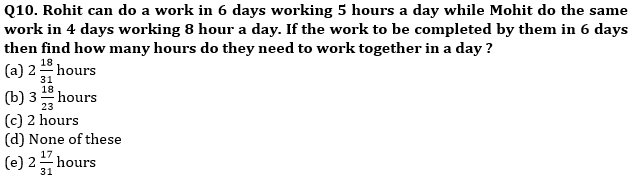Q11. Ramesh and Suresh can do a work in 18 days and 12 days respectively. Both are working together for x days and the Suresh left the work and Ramesh can do rest of the work in 8 days. Find the value of x is ?
(a) 6 days
(b) 4 days
(c) 8 days
(d) 12 days
(e) 10 days

Q12. Anurag and Vijay can finish a work in 8 days. If Anurag increased his efficiency by 100% of his initial efficiency and Vijay decreased his efficiency by 66⅔% of his initial efficiency then they together finish the same work in 6 days. In how many days Vijay alone can finish the same work?
(a) 25 days
(b) 40 days
(c) 20 days
(d) 35 days
(e) 22 days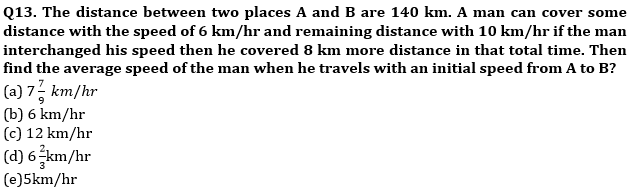Q14. There are 10 pens and 6 pencils in a box in which three are defective. Find the probability that one of them is pencil and two are pen ?
(a) 1/2
(b) None of these
(c) 27/57
(d) 27/56
(e) 3/5

Q15. How many 3 digit number can be formed by 0, 1, 2, 5, 7, 6, 9. Which is divisible by 5 and none of digit is repeated ?
(a) 55
(b) 58
(c) 68
(d) 54
(e) 52

Solutions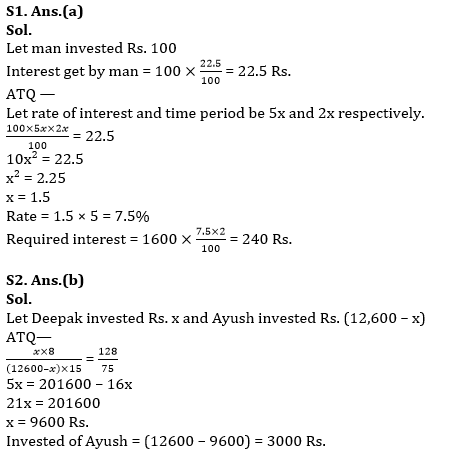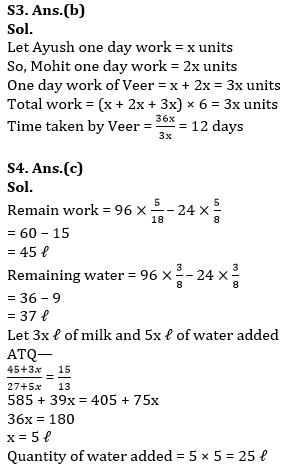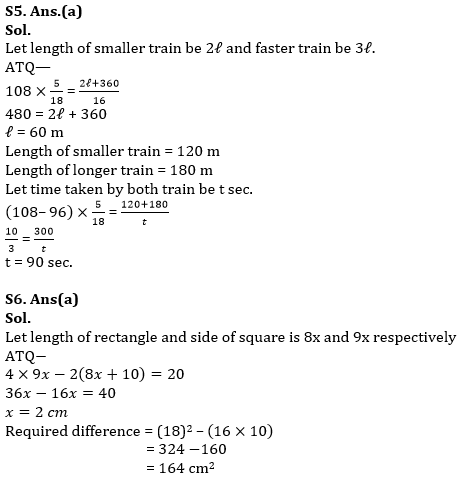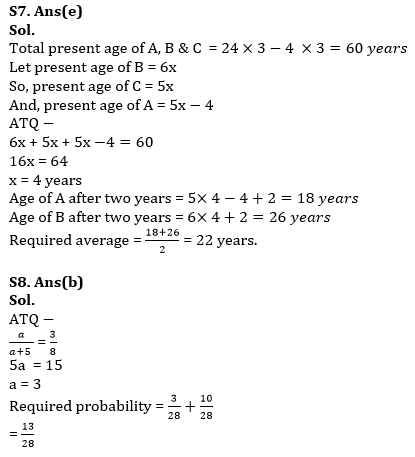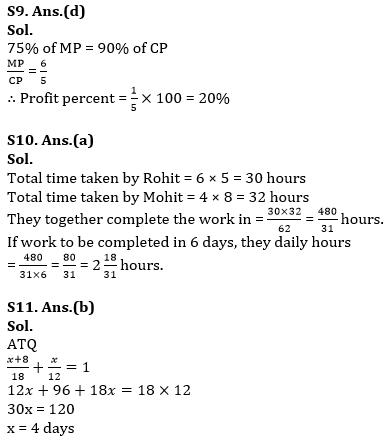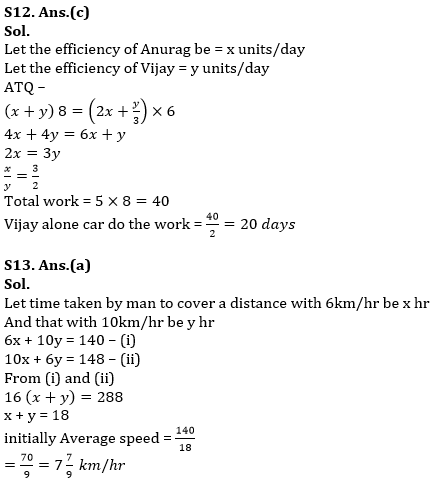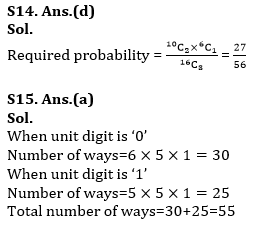#### Congratulations!Union Budget 2023-24: Free PDF